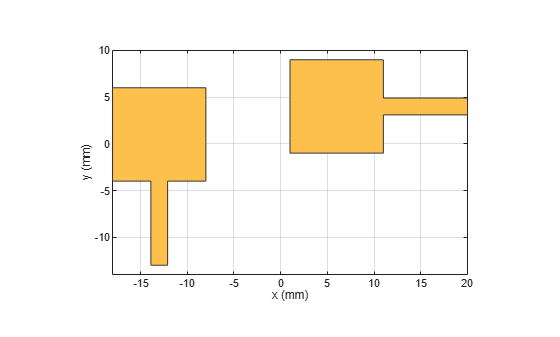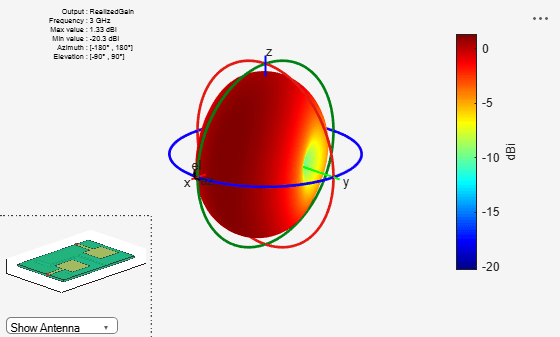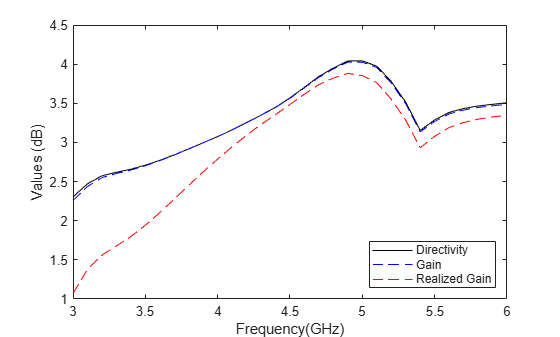# Design and Analysis of Compact Ultra-Wideband MIMO Antenna Array

This example shows the design and analysis of a compact ultra-wideband (UWB) Multiple-Input Multiple-Output (MIMO) antenna array as referred in . The American Federal Communications Commission (FCC) allowed commercial use in the 3.1 GHz to 10.6 GHz frequency range from the year 2002. Since then, there has been a significant amount of research done to develop antenna technology for this ultra-wide frequency range. The main challenge in designing a UWB antenna with such wide bandwidth is the multipath fading. A popular solution to overcome this fading effect is to use the MIMO antenna array technology which also increases the channel capacity of UWB systems.

### Create Upper Patches of Monopole Antennas

The MIMO array consists of two orthogonally placed planar monopole antennas lying in the horizontal plane. The size of the upper conductor of each monopole antenna is 10 mm by 16 mm. The dimension of the conductor backed dielectric substrate is 40 mm by 26 mm. The square radiator of the first monopole is at an offset of (7 mm, 14 mm, 0 mm) from one of the corners of the substrate. The second monopole's radiator is at an offset of (14 mm, 9 mm, 0 mm) from the diagonally opposite corner of the substrate.

```% Specify the geometry dimensions in m % The dimension of the square patch of each monopole radiator Lp=10e-3; % The length of the substrate along X-axis Xg=40e-3; % The width of the substrate along Y-axis Yg=26e-3; % The offset of the first monopole radiator px1=-Xg/2+7e-3; py1=-Yg/2+14e-3; % Lower left patch patch1 = antenna.Rectangle('Center',[0,0],'Length',Lp,'Width',Lp,'Center',[px1, py1]); % The offset of the second monopole radiator px2=Xg/2-9e-3-Lp/2; py2=Yg/2-8.1e-3-0.9e-3; % Upper right patch patch2 = antenna.Rectangle('Center',[0,0],'Length',Lp,'Width',Lp,'Center',[px2, py2]);```

### Create Feed Lines of Monopole Antennas

Feed both monopole antennas using two orthogonally oriented identical planar feedlines with length and width of 9 mm and 1.8 mm, respectively.

```% Upper left patch feedline feed1 = antenna.Rectangle('Center',[0,0],'Length',1.8e-3,'Width',9e-3,'Center',[px1, py1-Lp/2-9e-3/2]); % Upper right patch feedline feed2 = antenna.Rectangle('Center',[0,0],'Length',9e-3,'Width',1.8e-3,'Center',[px2+Lp/2+9e-3/2, py2]);```

### Create and Visualize Upper Layer

Join the patches to create the upper layer of the MIMO array which consists of two square radiators and two feed lines of the monopole antennas. Use the `show` function to visualize the uppermost layer of the array.

```% Join four components patchUpper=patch1+patch2+feed1+feed2; figure; show(patchUpper)```### Create and Visualize Ground Layer

The ground layer consists of two rectangular shapes, two rectangular slots and three stubs. The purpose of introducing such perturbation in the ground plane is to achieve good impedance matching across the entire UWB band and to minimize the mutual coupling between the two monopole antennas of the MIMO array.

```% Create left ground plane % Left rectangular ground block ground1 = antenna.Rectangle('Center',[0,0],'Length',29e-3,'Width',8e-3,'Center',[-Xg/2+29e-3/2, -Yg/2+4e-3]); % Slot in the left rectangular ground block groundcut1 = antenna.Rectangle('Center',[0,0],'Length',4e-3,'Width',1e-3,... 'Center',[-Xg/2+5e-3+4e-3/2, -Yg/2+8e-3-1e-3/2]); % Subtract the slot from the ground in the left ground block ground1=ground1-groundcut1; % Create right ground plane % Right rectangular ground block ground2 = antenna.Rectangle('Center',[0,0],'Length',8e-3,'Width',26e-3,'Center',[Xg/2-4e-3, 0]); % Slot in the right rectangular ground block groundcut2 = antenna.Rectangle('Center',[0,0],'Length',1e-3,'Width',4e-3,'Center',[Xg/2-8e-3+1e-3/2, py2]); % Subtract the slot from the ground in the right ground block ground2=ground2-groundcut2; % Ground interconnect ground3 = antenna.Rectangle('Center',[0,0],'Length',3e-3,'Width',1e-3,'Center',[Xg/2-8e-3-3e-3/2, -Yg/2+1e-3/2]); % Connect the three ground blocks ground=ground1+ground2+ground3; % First ground stub groundstub1= antenna.Rectangle('Center',[0,0],'Length',1e-3,'Width',18e-3,... 'Center',[-Xg/2+14e-3+1e-3/2, Yg/2-18e-3/2]); % Second ground stub groundstub2= antenna.Rectangle('Center',[0,0],'Length',5e-3,'Width',1e-3,'Center',[-Xg/2+14e-3-5e-3/2, Yg/2-1e-3/2]); % Third ground stub groundstub3= antenna.Rectangle('Center',[0,0],'Length',16e-3,'Width',1e-3,... 'Center',[Xg/2-8e-3+1e-3-16e-3/2, Yg/2-16e-3-1e-3/2]); % Connect all stubs with the ground block ground=ground+groundstub1+groundstub2+groundstub3; figure; show(ground)```### Assign Dielectric Properties

Design the entire MIMO array on a dielectric substrate with the relative permittivity and loss tangent values of 3.5 and 0.004, respectively. The thickness of the dielectric substrate is assumed to be 0.8 mm.

```% Dielectric d = dielectric; d.EpsilonR = 3.5; d.LossTangent=0.004; h=0.8e-3;```

### Create pcbStack Object

Create a `pcbStack` object using the same dielectric substrate. Assign `patchUpper` and `ground` to the upper and bottom layers of the `pcbStack` object, respectively.

```ps=pcbStack; ps.BoardThickness=h; r1bottom=antenna.Rectangle('Center',[0,0],'Length',Xg,'Width',Yg); ps.BoardShape =r1bottom; ps.Layers = {patchUpper,d,ground};```

### Excite Both Antennas

Define feed locations at the edge of the feedline of each antenna. Set the feed diameter manually to half of the feedline width. Excite each antenna with a unit amplitude and zero phase voltage source.

```% Feed location of the first antenna fx1=-Xg/2+6.1e-3+1.8e-3/2; fy1=-Yg/2; % Feed location of the second antenna fx2=Xg/2; fy2=Yg/2-8.1e-3-1.8e-3/2; % Assign excitation to both feed locations ps.FeedLocations=[fx1 fy1 1 3; fx2 fy2 1 3]; ps.FeedDiameter=1.8e-3/2;% in m ps.FeedVoltage=[1 1];% in Volt ps.FeedPhase=[0 0];% in degree```

### Mesh Geometry

Manually mesh the array geometry with the maximum edge length of 1.8 mm.

```ax=figure; mesh(ps,'MaxEdgeLength',1.8e-3)```### Visualize S-parameters of Array

Use the `sparameters` function to calculate the scattering parameters of the array. As it is a two-port array, it has four S-parameters, among which the S11 and S22 represent the reflection coefficient at the port 1 and 2, respectively. The mutual coupling between two ports is characterized by S12 and S21. Use the `rfplot` function to visualize the port parameters. Due to the large number of basis functions, simulation of the frequency variation can take several minutes. By default, simulation of the frequency variation is disabled and a precomputed model is used. To enable the simulation of the frequency variation, set `runModel` to true.

```% Define the frequency range in Hz f1=linspace(3,6,31)*1e9; runModel = false; if runModel % Compute the S-parameters s=sparameters(ps,f1); else % Load precomputed data if runModel is set to false load('frequencyVariationData.mat') end % Plot the s-parameters figure; rfplot(s)```### Plot Directivity, Gain, and Realized Gain Patterns with Both Excitations

Use the `pattern` function to visualize different radiation patterns of the array with excitation in both antennas. The input argument `'Type'` of pattern will determine whether it computes directivity, gain or realized gain pattern. By default, `'Type'` is `'directivity'` for a lossless antenna and `'gain'` for a lossy antenna. The directivity is a ratio of the radiation intensity in a specific angular direction to the average radiated power. It does not depend on material loss or impedance mismatch. The gain is a product of the directivity and radiation efficiency. The radiation efficiency is the ratio of the total radiated power to the total input power going into the antenna's/array's input port(/s). The material losses like finite conduction loss and dielectric loss are accounted in the radiation efficiency and gain parameter. As a part of a complete RF system, antenna/array is commonly connected with an impedance matching network. The impedance mismatch of the antenna or the array with such impedance network leads to finite port mismatch loss which is characterized by the port efficiency. Port efficiency is a ratio of the input power provided by the external excitation port(/s) and the power received at the antenna's/array's input feeding edge(/s). The multiplication of gain with the port efficiency provides the realized gain. The directivity, gain, and realized gain patterns of the MIMO array at 3 GHz are shown below.

```figure; pattern(ps,3e9,'Type','directivity');``````figure; pattern(ps,3e9,'Type','gain');``````figure; pattern(ps,3e9,'Type','realizedgain');```Comparison of the radiation patterns shows that the gain is lower than the directivity as the radiation efficiency is less than 1. Similarly, the realized gain is lower than the gain value due to finite impedance mismatch loss. Use the `efficiency` function to calculate the radiation efficiency of the array.

`Efficiency=efficiency(ps,3e9);`

### Visualize Directivity, Gain, and Realized Gain Patterns with Single Excitation

Similar to , if only one antenna of the array is excited and the other is shorted, a corresponding excitation model is introduced through the `FeedVoltage` and `FeedPhase`. Only the first antenna is assumed to be excited here.

```ps.FeedVoltage=[1 0]; ps.FeedPhase=[0 0];```

Compute and plot the directivity, gain, and realized gain values over a broad frequency range.

```if runModel % Compute directivity, gain, and realized gain for m=1:length(f1) patD1=pattern(ps,f1(m),'Type','directivity'); patG1=pattern(ps,f1(m),'Type','gain'); patRG1=pattern(ps,f1(m),'Type','realizedgain'); D1(1,m)=max(max(patD1)); G1(1,m)=max(max(patG1)); RG1(1,m)=max(max(patRG1)); end end % Visualize the direcitivity, gain, and realized gain patterns figure; plot(f1./1e9,D1,'k') hold on plot(f1./1e9,G1,'--b') plot(f1./1e9,RG1,'--r') xlabel('Frequency(GHz)') ylabel('Values in (dB)') legend('Directivity', 'Gain', 'Realized Gain')```The gain values are observed to increase with the frequency. This indicates that the array radiation pattern becomes more directional as frequency increases.

### Conclusion

This example shows how to efficiently design any advanced complex PCB antenna array using Antenna Toolbox™ and evaluate its impedance and radiation characteristics using Method of Moment (MoM) solver based full-wave simulation.

### References

 L. Liu, S. W. Cheung, T. I. Yuk and D. Wu. "A Compact Ultrawideband MIMO Antenna," 2013 7th European Conference on Antennas and Propagation (EuCAP), Sweden, 2013.